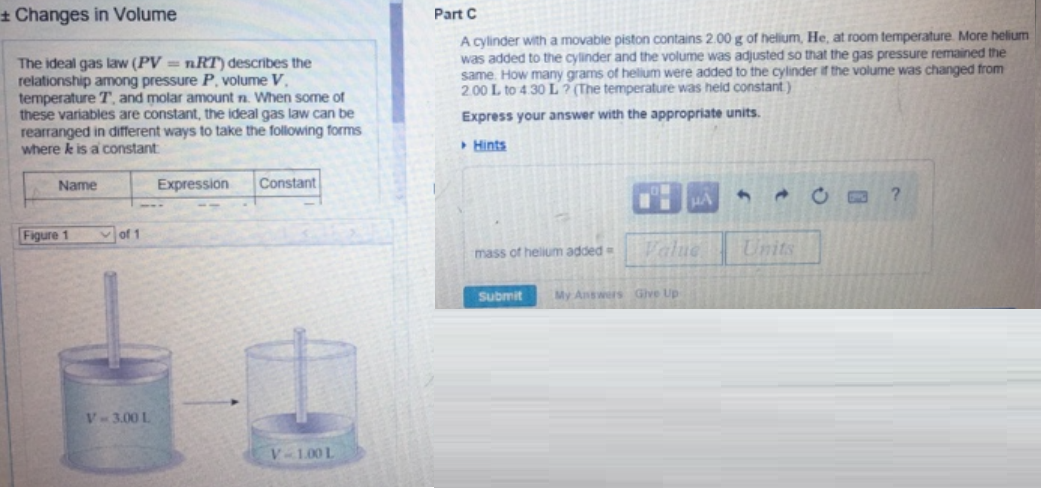# Solution: The ideal gas law (PV = nRT) describes the relationship among pressure P. volume V, temperature T. and molar amount n. When some of these variables are constant, the ideal gas law can be rearranged in different ways to take the following forms where k is a constant. Part CA cylinder with a movable piston contains 2.00 g of helium. He at room temperature. More helium was added to the a under and the volume was adjusted so that the gas pressure remained the same. How many grams of helium were added to the cylinder if the volume was changed from 2.00 L to 4.30 L? (The temperature was held constant) Express your answer with the appropriate units.

###### Problem

The ideal gas law (PV = nRT) describes the relationship among pressure P. volume V, temperature T. and molar amount n. When some of these variables are constant, the ideal gas law can be rearranged in different ways to take the following forms where k is a constant.

Part C

A cylinder with a movable piston contains 2.00 g of helium. He at room temperature. More helium was added to the a under and the volume was adjusted so that the gas pressure remained the same. How many grams of helium were added to the cylinder if the volume was changed from 2.00 L to 4.30 L? (The temperature was held constant)View Complete Written Solution